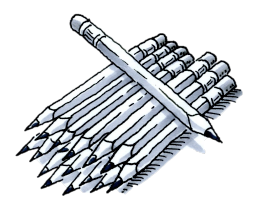### Home > MC2 > Chapter 10 > Lesson 10.2.1 > Problem10-88

10-88.

Juan found that $20$ new pencils weigh $12$ ounces. How much will $50$ new pencils weigh? Show your reasoning.

Notice that this is a proportion problem. The weight per pencil does not change as the number of pencils increases or decreases.

Set up equivalent ratios that compare the number of pencils to the weight of the pencils.

$\frac{20\;\text{pencils}}{12\;\text{ounces}} = \frac{50\; \text{pencils}}{\it x}$

Solve for $x$.

$x = 30$ ounces